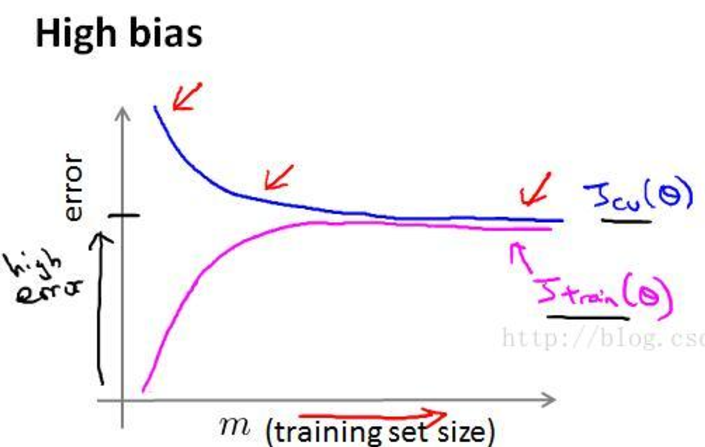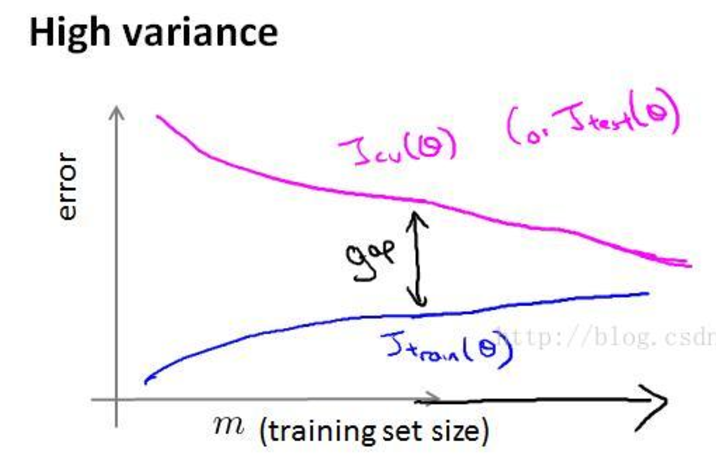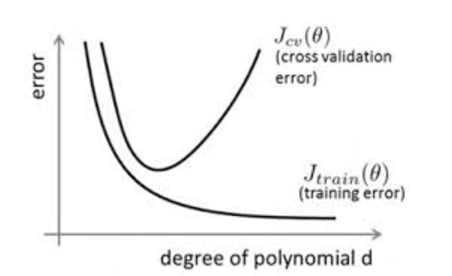35 篇文章 0 订阅

# 过拟合与数据不平衡

## 为什么会过拟合？

1. 对于数据样本，可能存在隐单元的表示不唯一，即产生分类的决策面不唯一，随着学习的进行，BP算法使权值可能收敛过于复杂的决策面。
2. 权值学习迭代次数足够多，拟合了训练数据中的噪声和训练样例中没有代表性的特征。

## 如何判断过拟合？

Jtrain(θ)=1mtraini=1mtrain(y^iyi)2(1) (1) J t r a i n ( θ ) = 1 m t r a i n ∑ i = 1 m t r a i n ( y ^ i − y i ) 2

Jval(θ)=1mvali=1mval(y^iyi)2(2) (2) J v a l ( θ ) = 1 m v a l ∑ i = 1 m v a l ( y ^ i − y i ) 2

Jtest(θ)=1mtesti=1mtest(y^iyi)2(3) (3) J t e s t ( θ ) = 1 m t e s t ∑ i = 1 m t e s t ( y ^ i − y i ) 2## 怎么预防过拟合？

1. 数据清洗
导致过拟合的一个原因就可能是数据不纯导致的，若是出现过拟合就需要考虑是否需要重新进行数据清洗。数据清洗的目的之一就是减少特征，留下具有代表性的特征。

2. 数据增强
在训练过程中真正使用的训练数据有点少，通通过数据增强来增加训练数据。常用的方法有：
<1> 从数据来源处采集更多的数据；
<2> 通过原有的数据作一些简单变化，如添加噪声等；
<3> 重采样；
<4> 估计当前数据的分布模型用以产生更多的数据。

3. 正则化
正则化参数$\lambda$$\lambda$的大小也会引起过拟合和欠拟合问题：过大的值调节能力太强易引发欠拟合；过小的值调节能力太弱易引发过拟合。

<0> L0 L 0 $L_0$正则项
L0 L 0 $L_0$正则是基于 L0 L 0 $L_0$范数的。指向量中非零元素的个数。

<1> L1 L 1 $L_1$正则项
L1 L 1 $L_1$正则是基于 L1 L 1 $L_1$范数的，即在代价函数之后添加 一个 L1 L 1 $L_1$范数和项。

C=C0+λi=1m|wi| C = C 0 + λ ∑ i = 1 m | w i |
其中 C0 C 0 $C_0$为原来的代价函数， λ λ $\lambda$为正则项系数。那么参数 w w $w$的基于梯度下降法的更新规则可以变为：
其中， α<0,β<0,sgn() α < 0 , β < 0 , s g n ( ⋅ ) $\alpha \lt 0,\beta \lt 0,sgn(\cdot)$为符号函数。从该式子可以看出，当 w w $w$在0附近波动时，正则项的存在会使得$w$$w$向0靠近。但是注意 L1 L 1 $L_1$范数在0点不可导，因此需要使用不含正则项的代价函数来更新权值 w w $w$

<2>${L}_{2}$$L_2$正则项
L2 L 2 $L_2$正则是基于 L2 L 2 $L_2$范数的，即在代价函数之后添加 一个 L2 L 2 $L_2$范数和项。

C=C0+λ2i=1mw2i C = C 0 + λ 2 ∑ i = 1 m w i 2
其中 C0 C 0 $C_0$为原来的代价函数， λ λ $\lambda$为正则项系数。那么参数 w w $w$的基于梯度下降法的更新规则可以变为：
$w:=w+\alpha \frac{\mathrm{\partial }{C}_{0}}{\mathrm{\partial }w}+\beta \lambda$
其中， α<0,β<0 α < 0 , β < 0 $\alpha \lt 0,\beta \lt 0$

总结：正则项的引入是为了降低模型的复杂度，从而避免过分拟合训练数据，包括噪声和异常点。从另一个角度来看，正则项的引入是假设模型服从先验概率，只是不同的正则项所代表的分布是不一样的。 L1 L 1 $L_1$是拉普拉斯先验而 L2 L 2 $L_2$是高斯先验，均服从均值为0，协方差为 1λ 1 λ $\frac 1\lambda$

• dropout
在著名的AlexNet卷积神经网络中就采用了该种方法。在神经网络的训练过程中通过使隐层的一些神经元在某些时候停止工作(休眠)而输入输出层神经元个数不变。

• early stopping
在模型的学习过程中，常常采用的迭代的方法来更新训练参数，比如常见的梯度下降算法。而early stoppoing 就是一种用来打断迭代过程的方法。

具体作法如下：在每一次遍历完所有的训练集数据后，计算验证集的精度，当其精度不在提高时(可以假设精度多少次迭代之内变化不超过一个阈值就认为不在变化；或者，记录最好的精度，之后多少代之内均未有更高的精度出现则认为不变了)就停止迭代。

• ## 数据不平衡

在机器学习使用的数据集中，不同种类的数据量可能相差很大，而我们往往比较关注其中那一部分量小的数据，这就是数据不平衡。当训练过程中使用不平衡的数据得到的模型并不是很准确。通常可以使用一下几种方法来检测异常类。

1）使用正确的评价指标
模型的评价指标有很多种，可以采用不同的指标来衡量，避免数据不平衡训练出不好的模型结果。
2）重新采用训练集
可以使用欠采样或者过采样来进行数据的重新采样。
3）正确使用K折交叉验证
使用过采样来平衡数据时，应当使用交叉验证。
4）组合不同重采样数据
用少数类的所有样本和多数类的n个不同样本训练多个模型然后进行组合。
5）用不同比例重新采样数据
可以使用不同的比例重采样数据集，然后训练多个模型。
6）聚类
可以换一种思路，减少数据量大的类别也可以起到平衡数据量的作用。常用的方法可以对多数类进行聚类，使用质心来替代这一类，这样就能减少多数类的数据量。
7）设计模型
可以设计适用于数据不平衡的模型，如XGBoost在模型内部对数据了很不错的处理。也可以通过设计一个损失函数来惩罚少数类的错误分类。

09-252万+07-011055
08-293
01-115555
04-072994
04-278730
08-283万+
08-051万+
06-09332
03-153333
11-14754
03-11457
04-08211
11-042万+
09-17
09-18点击重新获取扫码支付余额充值